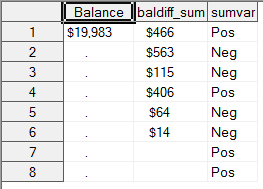## Difficulty setting a dynamic numeric value with sgrender waterfall

My version is SAS 9.3. The code includes a dynamic variable in a sgrender waterfall graph. It appears the sgrender statement does not recognize the dynamic value for the initialbarvalue as a number when I use the dynamic variable - the message in the log states "INITIALBARVALUE=balance is invalid. The value cannot be converted to a number. The default will be used" and as a result the render statement sets the initialbar value to zero. If I replace the sgrender statement with a constant number it works perfectly. I did try lineraropts tickvaluelist to set the yaxis range but that wasn't successful.

Here is the code:

proc template;

define statgraph waterfall;

dynamic _val;

begingraph  /

designheight = 600 designwidth = 1200 border = false backgroundcolor=white pad=(right=5%);

discreteattrmap name="colorbysign";

value 'Pos' / fillattrs=(color=ForestGreen);

value 'Neg' / fillattrs=(color=Red);

value 'x' / fillattrs=(color=LightBlue);

enddiscreteattrmap;

discreteattrvar attrvar=signvar var=sumvar attrmap="colorbysign";

entrytitle _id='title' halign=left 'Net Change in Loan Balance by Transactions (in Millions)' /

textattrs=(color=darkblue weight=bold style=normal size=14 family='Arial');

layout overlay/

xaxisopts = (display=(tickvalues) griddisplay=on gridattrs=(pattern=dot)

/*labelattrs=(color=white weight=bold size=12 family='Arial')*/

tickvalueattrs=(size=12pt weight=bold color=darkblue))

yaxisopts = (display=none griddisplay=on gridattrs=(pattern=dot)

/*linearopts=(tickvaluelist=(19000 19500 20000 20500 21000 21500 22000))*/

tickvalueattrs=(size=12pt weight=bold)

labelattrs=(color=white weight=bold size=12 family='Arial'));

waterfallchart      category=type response=baldiff_sum/ rolename=(tip1=type) tip=(tip1)

colorgroup=signvar barlabel=true datalabel=Transactions

rolename=(tip1=type) tip=(tip1)

finalbarattrs=(color=grey)

initialbarattrs=(color=lightblue)

initialbartickvalue="Start"

initialbarvalue=_val baselineintercept=18500000000

barlabelattrs=(color=darkblue weight=bold family='Arial' size=11);

endlayout;

endgraph;

end;

title ;

proc sgrender template=waterfall data=combine3;

dynamic _val='balance';

run;

1 ACCEPTED SOLUTION

Accepted Solutions

## Re: Difficulty setting a dynamic numeric value with sgrender waterfall

If you only have on actual value for the variable in the input data set then something like:

proc sql noprint;

select max(balance) into : mb

from combine3;

quit;

proc sgrender template=waterfall data=combine3;

dynamic _val=&mb;

run;

might work.

8 REPLIES 8

## Re: Difficulty setting a dynamic numeric value with sgrender waterfall

You  might look at two things: 1) is variable balance in combine3 actually numeric or a character variable impersonating a numeric

2) what is the first value of balance in combine3? it may not like a missing value.

## Re: Difficulty setting a dynamic numeric value with sgrender waterfall

Thank you BallardW,

The balance field is numeric.

I used proc tab to merge two files to create the following dataset. First column is my start balance 2nd column is the various walk categries - thrird indicates balance direction for the walk. As you see, the first column first row is the balance I want for the intialbarvalue. I triple checked and the balance value is numeric.Thanks for any help and ideas you can share## Re: Difficulty setting a dynamic numeric value with sgrender waterfall

Does the code work passing a numeric value explicitly?

proc sgrender template=waterfall data=combine3;

dynamic _val=19983;

run;

I expect it is trying to set the initial value to "balance" the string not the vale of the variable.

I'm not sure if a dataset variable value will work in this context as written. Since the variable could change for each record to be charted it wouldn't quite be initial for the whole series.

## Re: Difficulty setting a dynamic numeric value with sgrender waterfall

Yes, it works fine passing a numeric value - no problem. And Right! The value has to be dynamic so that it automaticaklly changes and the initial value changes.Jay54
Meteorite | Level 14

## Re: Difficulty setting a dynamic numeric value with sgrender waterfall

Is this issue resolved?  If not, can you attach a full runnable program with data so we can see what is going on. Which release of SAS are you using?

## Re: Difficulty setting a dynamic numeric value with sgrender waterfall

If you only have on actual value for the variable in the input data set then something like:

proc sql noprint;

select max(balance) into : mb

from combine3;

quit;

proc sgrender template=waterfall data=combine3;

dynamic _val=&mb;

run;

might work.

## Re: Difficulty setting a dynamic numeric value with sgrender waterfall

BallardW, that was perfect. Now I can figure out how to make the baselineintercept variable (i.e. 80% of the balance variable). I really appreciate everyone's comments. This is a great community.## Re: Difficulty setting a dynamic numeric value with sgrender waterfall

Numeric values typically align to the right even when formatted as currency, text aligns to the left. Your values are aligned to the left indicating a strong possibility it is character.

Can you post the proc contents for your variable?

Discussion stats
• 8 replies
• 1148 views
• 3 likes
• 4 in conversation